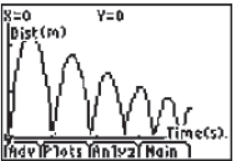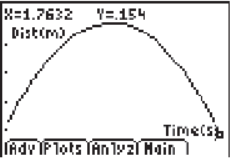# Activities

••• ##### Subject Area

• Math: Algebra I: Quadratic Functions
• Science: Physics: Kinematics

• ##### Author9-12

60 Minutes

• ##### Device
• TI-83 Plus Family
• TI-84 Plus
• TI-84 Plus Silver Edition
•TI-84 Plus C Silver Edition
•TI-84 Plus CE
•TI-84 Plus CE Python
• ##### Software

TI Connect™
TI Connect™ CE

• ##### Accessories

CBR™/CBR 2™
TI Connectivity Cable

• ##### Other Materials
This is Activity 12 from the EXPLORATIONS Book:
EasyData Activities: Modeling Algebraic Functions with Data Collection Activities.

The following materials are required for this activity:
• Bouncing ball

## Bouncing Ball#### Activity Overview

In this experiment, students collect the height versus time data of a bouncing ball using the CBR 2™. This activity investigates the values of height, time, and the coefficient A in the quadratic equation, Y = A(X - H)2 + K, which describes the behavior of a bouncing ball. They graph scatter plots, graph and interpret a quadratic function, apply the vertex form of a quadratic equation, and calculate the maximum value of a parabola.

#### Key Steps

•Students use a ball and a CBR2 to collect data as the ball bounces using the EasyData App.

•Students are directed to select one parabolic region to analyze with the Quadratic Fit function in the EasyData App’s Anlyz menu, then the Quadratic Regression command in the CALC menu and finally using the vertex form from the Home Screen and Y= Menu.